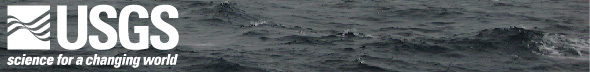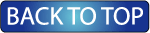Oceanographic Observations, Hudson Shelf Valley, U.S. Geological Survey Open-File Report 02-217

## Matlab m-Files

Most of the plots and statistical analysis included with this report were created using Matlab software created by Mathworks (www.mathworks.com). Plots were further aesthetically modified and converted to tagged PDF version using Adobe (www.adobe.com) Illustrator and Acrobat.

DISCLAIMER: Matlab m-files provided here were not created as turn key programs. They were designed to run on Matlab 6.0 with NetCDF installed. Users are encouraged to look at (crusty.er.usgs.gov/~cdenham/) for more information on the NetCDF format. M-files are provided here merely to give the user a better understanding of techniques used, and are not supported by their creator or by USGS. Additional sub-routines may be found at:
1) crusty.er.usgs.gov/~cdenham/snackbar/snackbar.html
2) sea-mat.whoi.edu/

### Plotting Tools Used for this Report:

ADCP_Plotmaker: Plots the low-passed wind stress for a chosen station along with an image plot of the available low-passed ADCP data in directional component form.

ADCP_Rot_Plotmaker: Plots the low-passed wind stress for a chosen valley station along with an image plot of the available low-passed ADCP data in directional component form, rotated along the valley axis.

ADCP_Rot_Valley: Creates image plots of the along valley axis component of available low-passed ADCP data for the valley stations.

ADCP_ScatterPlot_NY: For a given station, creates a four paneled plot containing scatter plots of eastward and northward current component and principal axes ellipse for hourly-averaged and low-passed ADCP current data from 5 meters below the surface and from the deepest depth bin available.

ADCP_Whisk_All_Plotmaker: Given a specific depth, or 'B' for the bottom-most bin, this program creates whisker plots of the low-passed ADCP data from each station closest to the given depth.

ADCP_Whisk_Plotmaker: Given a specific station, this program creates whisker plots of the low-passed wind stress and ADCP data from that station at particular depths (5, 15, and bottom for the shelf station; 5, 15, 30, 45, and bottom for the valley stations).

ADCP_Whisk_Rot_Val_Plotmaker: Given a specific depth, or 'B' for the bottom-most bin, this program creates whisker plots of the low-passed ADCP data rotated to along and across valley axis from each of the valley stations (A, B, C, & F) at approximately the selected depth.

ADCP_Whisk_Shelf_Plotmaker: Given a specific depth, or 'B' for the bottom-most bin, this program creates whisker plots of the low-passed ADCP data from each of the shelf stations (D & E) closest to the selected depth

ADCP_Whisk_Valley_Plotmaker: Given a specific depth, or 'B' for the bottom-most bin, this program creates whisker plots of the low-passed ADCP data from each of the valley stations (A, B, C, & F) closest to the selected depth

All_Sal_Temp_Plotmaker: Program which creates four separate plots, one each of deep and shallow salinity and deep and shallow temperature from each of the stations.

animateNY: Program that creates the individual frames (saved as PPM files) which can be combined to create the animation of the New York data in this data report.

contourEnCTD: Program that creates contour plots of the temperature, salinity, fluorescence, and beam attenuation data from the Endeavor cruise casts as a function of depth and distance along the canyon.

contourOcCTD: Program that creates contour plots of the temperature, salinity, fluorescence, and beam attenuation data from the Oceanus cruise casts as a function of depth and distance along the canyon.

Curr_Scat_Plot: For a given station, creates a multi-paneled plot containing scatter plots of eastward and northward current component and principal axes ellipse for hourly-averaged and low-passed BASS/MAVS current data from the available depths.

Curr_Sp_Dir_Plotmaker: Program which sorts current data for a given station and a given depth into current speed and direction bins and plots those bins.

locsCTD: Program which creates a map of the New York coast line and bathymetry in the area of the New York Bight along with the cast locations from the Endeavor and Oceanus cruises.

Mean_Flow_Ell_BASS: Program to plot the low passed wind stress and mean flow (by month) for shelf (plot one) and valley (plot two) stations from the BASS data.

Mean_Flow_Ellipse_Plot: Program to plot the low passed wind stress and mean flow (by month) for each station at specific depths from ADCP data.

NYCurr_Whisk: Program to plot ADCP current data as whiskers off of the x-axis. Had to be specifically tailored to site/data type because of axes range and labeling restrictions.

OBS_ATTN_Plotmaker: Given a specific station (other than F, at which there was no OBS or ATTN instrumentation), this program plots optical backscatter and beam attenuation from available depths.

P_CS_OBS_Plotmaker: Given a specific station, this program plots the st. deviation of pressure, and the current speed and optical backscatter from the available depths.

plotEnCTD: Program which takes the CTD data from the Endeavor cruise and creates plots of certain data variables for each cast.

plotMeteorNY: Program which creates time series plots of several environmental variables from the Ambrose Light station.

plotMeteorStatsNY: Program which plots meteorological statistics from various buoys.

plotOcCTD: Program which takes the CTD data from the Oceanus cruise and creates plots of certain data variables for each cast.

plotOpt2Sed: Program which plots beam attenuation and optical backscatter vs. sediment concentration for Oceanus and Endeavor CTD data.

PlotStatsNY: Program which plots the statistics of current and temperature calculated with Stats_Curr_Temp.m

PressurePlot.m: Given a specific station, this program plots the low-passed wind stress, the hourly averaged pressure, and the low-passed pressure.

Sal_Plotmaker: Given a specific station, this program plots the salinity data from the surface, two intermediate depths (if available), and the bottom.

Temp_Plotmaker: Given a specific station, this program plots the temperature data from the surface, two intermediate depths (if available), and the bottom.

whiskWindNY: Program to plot wind stress data as whiskers off of the x-axis. Had to be specifically tailored to site/data type because of axes range and labeling restrictions.

### Statistics Calculation Tools:

Ellipse_Calc: Program which calculates the ellipse parameters for a given station at a given depth and outputs on-screen in matlab.

meteorStatsNY: Program to calculate various meteorological statistics for a given buoy.

nanmax: Program to calculate the maximum of a set of values ignoring the matlab fill value of "NaN".

nanmean: Program to calculate the mean of a set of values ignoring the matlab fill value of "NaN".

nanmin: Program to calculate the minimum of a set of values ignoring the matlab fill value of "NaN".

nanstd: Program to calculate the standard deviation of a set of values ignoring the matlab fill value of "NaN".

nansum: Program to calculate the sum of a set of values ignoring the matlab fill value of "NaN".

nanvar: Program to calculate the variance of a set of values ignoring the matlab fill value of "NaN".

Stats_Curr_Temp: Program which calculates the mean, maximum, minimum, and standard deviation values of current and temperature, by month and for the entire data set, at a given station and depth. Calls PlotStatsNY to plot these results.

Stats_Current: Program which calculates a variety of statistics for currents from several ADCP depths and all available VMCM/BASS data and outputs it to the screen. For the data report, this information was recorded in a table.

Stats_Ellipse: Program which calculates the ellipse parameters for all available VMCM/BASS data from a given station and from several ADCP depths and outputs it to the screen. For the data report, this information was recorded in a table.

### Other Tools Used:

angleXtoCompass: Program to convert polar coordinate angles (measured counter-clockwise from x-axis) to compass angles (measured clockwise from north)

binsort: Program which sorts a given variable into bins defined by two vectors.

gregaxd_fooled: Program modified from gregaxd which can handle labeling the time axis when it has been scaled by some factor.

gregaxdNM: Modification of gregaxd which does not include month labels.

NY_Tides: Computes a tidal analysis on the pressure data available from a given New York station.

NY_Tides_Curr: Computes a tidal analysis on the current data available from a particular depth at a given New York station.

pcaben: Program which calculates ellipse parameters for currents.

ridfill: Program which replaces fill values with zeroes.

ridfill_nan: Program which replaces fill values with "NaN's" (Not A Number - matlab's fill value)

singleJD: This program converts julian dates from a two vector format to a single vector format.

transformNY: Given an angle of rotation for the valley, this program rotates current data to along and across valley axis.

UVtoSpDir: This program converts u and v (eastward and northward) velocity components into speed and direction (direction is standard polar coordinate, measured counter-clockwise from x-axis)

value2Index: Given a list of values and a target value, this program finds the index of the value in the list closest to the target value.

varify: Program which excludes empty data points from a data set.